# Converted-Phase Seismic Imaging: Amplitude

## Transcription

Converted-Phase Seismic Imaging: Amplitude
```Converted-Phase Seismic Imaging: Amplitude-Balancing
Source-Independent Imaging Conditions
Andrey H. Shabelansky1 , Alison Malcolm2 , and Michael Fehler1
1 Earth Resources Laboratory,
Department of Earth, Atmospheric and Planetary Sciences, Massachusetts
Institute of Technology
2 Earth
Sciences Department, Memorial University of Newfoundland
Abstract
We present cross-correlational and de-convolutional forms of a source-independent converted-phase
imaging condition (SICP-IC) and show comparisons between them using the synthetic Marmousi model.
The results show significant improvements in spatial resolution and amplitude-balancing with the deconvolutional forms. This opens up the possibility of true-amplitude full-wavefield imaging with enormous advantages in processing time and cost by using source-independent imaging compared to conventional source-dependent imaging.
Introduction
Seismic imaging of the earth’s interior is of a great importance in exploration and global seismology.
It produces images of subsurface discontinuities associated with impedance contrasts through reflection, transmission or conversion coefficients of propagating waves. One of the pioneering studies on
seismic imaging was presented by Claerbout (1971) where the concept of imaging condition (IC) was
introduced. This concept is based on a fundamental assumption that acquisition/survey geometry is well
known: both source and receiver locations are known and seismic waves can be propagated from these
locations. However, when source information is not available, seismic images cannot be constructed
using Claerbout’s approach. An alternative approach is to form an image using the interference between
different wave types propagated backward in time from receiver locations only (e.g., Shang et al., 2012;
Shabelansky et al., 2014). We call this imaging condition Source-Independent Converted-Phase Imaging
Condition (SICP-IC). In this paper, we present amplitude-balancing SICP-ICs and test them numerically
with synthetic Marmousi model.
Source independent converted phase imaging condition (SICP-IC)
Propagating elastic waves from a point source at the surface generates, at the reflection point, both reflected and converted phase waves (see Figure 1(a)). Claerbout’s imaging condition attempts to mimic
f l inc
re f l inc
the reflection coefficient R pp = ure
pp /u p (or alternatively the conversion coefficient R ps = u ps /u p ) of
the reflection point, where the incident wavefield, uinc
p , is calculated by forward propagation from the
fl
source and is often called the source wavefield, and the reflected wavefield ure
pp (or converted wavefield
fl
ure
ps ) is calculated by back-propagation in time from the receivers and is called the receiver wavefield
(see Figure 1(b)). By contrast, SICP imaging uses two back-propagated wavefields (i.e., PP and PS,
and/or SS and SP) simultaneously (see Figure 1(c)), and it turns out that the SICP-IC mimics the ratio
between reflection and conversion (or conversion and reflection) coefficients, which we call the conversion ratio coefficients C (Shabelansky, 2015)
re f l
R ps
=
Cps :=
R pp
u ps
uinc
p
re f l
u pp
uinc
p
re f l
fl
ure
ps
=
,
re f l
u pp
Rsp
Csp :=
=
Rss
usp
uinc
s
re f l
uss
uinc
s
=
fl
ure
sp
fl
ure
ss
,
(1)
where Cps and Csp are given for incident P- and S-wavefields respectively. Note that the incident wavefield cancels in equation 1, and the source location, marked with the star in Figure 1(c), is not used in
SICP-IC (i.e., the incident wavefield marked with a grey line is not used). Moreover, the source location
of the signal can be anywhere along the grey line (see Figure 1(d)), which makes SICP-IC applicable
to any type of seismic data (i.e., active and passive). In addition, the sources along these grey lines
can be outside of the computational grid while still constructing the image only in the vicinity of the
receivers. This is the strength of this method. The explicit form of the SICP-IC for Ns sources with
P-wave illumination (i.e., denominator) is
Ipdecon (x)
Ns
:= ∑
j
Z 0 u j (x,t) · u j (x,t)
ip
is
T
j
(uip
(x,t))2 + ε 2
dt,
(2)
dt,
(3)
and with S-wave illumination
Isdecon (x)
Ns
:= ∑
j
Z 0 u j (x,t) · u j (x,t)
ip
is
T
(uisj (x,t))2 + ε 2
where I is the calculated image and ε 2 is a small number. The subscript i can be either p or s. The
spatial and time coordinates are x and t, and T is the maximum recorded time; it is at the lower limit of
the integral (i.e., the data are propagated backward in time). The superscript j refers to the source index,
and the subscript of I denotes the illuminating wavefield. The vector wavefields uip and uis refer to Pand S-wavefields, regardless of the wave type of their incident wavefield, and are calculated as
uip (x,t) = ∇∇ · u(x,t),
uis (x,t) = −∇ × ∇ × u(x,t),
77th EAGE Conference & Exhibition 2015
IFEMA Madrid, Spain, 1–4 June 2015
(4)
Source
Receivers
Source
Receivers
Surface
Pinc
Source
Surface
PPrefl
PSrefl
PSrefl
Pinc
Receivers
Surface
Reflector
PPrefl
Pinc
PSrefl
Reflector
Reflection
point
Image
point
(a)
(b)
PPrefl
Reflector
Image
point
(c)
Receivers
Surface
Source
Sinc
?
iS
iP
Pinc
Reflector
Image
point
Pinc
Sinc
Source
?
(d)
Figure 1 Schematics illustrating (a) elastic wave propagation that samples a point (blue dot) on a
reflector with incident P-wavefield, (b) imaging of the reflection point using Claerbout’s IC, and (c)
SICP-IC. (d) Generalization of SICP-IC for reflection and transmission seismic data with either (or
both) incident P- or/and S-wavefield(s). The red arrows in (b), (c) and (d) indicate the direction of the
propagating waves that form an image. The grey lines mark available wave types that are not used in the
image construction. Although source information indicates the origin of the waves, the image obtained
with SICP-IC uses receiver information only.
where u is the total displacement (or particle velocity), and ∇, ∇· and ∇× are gradient, divergence and
curl operators, respectively. The form of the IC given in equations 2 and 3 is called a de-convolutional
IC, denoted with the superscript decon. This IC depends strongly on the choice of the ε 2 , which changes
for different data sets and may still be unstable. As an alternative to the de-convolutional IC, the crosscorrelational IC is defined by taking only the numerator of equations 2 and 3 (Shabelansky et al., 2014)
Ns
Z 0
j
T
I cross (x) = ∑
uip (x,t) · uis (x,t)dt.
(5)
Note that the cross-correlational SICP-IC is unconditionally stable. However, because the denominator
is omitted, the cross-correlational image is not-amplitude balanced.
The de-convolutional ICs, presented in equations 2 and 3, go to infinity when the wavefield of the
denominator is zero. This contradicts the idea behind the IC: when one wavefield is zero the image
should be zero. Another downside of the IC in equations 2 and 3 is that only one wavefield is used
for normalization/illumination (either P- or S-wave but not both). To alleviate these two limitations, we
propose another IC that will be set to zero when one of the waves is zero and will be between -1 and 1:
the values of ±1 are when both wavefields are equal in amplitude with the same or opposite sign. We
call this the normalized de-convolutional SICP-IC. The explicit form of this normalized SICP-IC is:
Ns
I M (x) := ∑
j
Z 0
T
(uip
4uip (x,t) · uis (x,t)
dt.
2
2
ip (x,t) · uis (x,t)| + (uis (x,t)) + ε
(x,t))2 + 2|u
77th EAGE Conference & Exhibition 2015
IFEMA Madrid, Spain, 1–4 June 2015
(6)
Numerical Tests - Marmousi Model
To examine the stability and illustrate the advantages of different SICP-ICs given in equations 2, 3, 5 and
6, we test them with the synthetic Marmousi model. All elastic wave solutions for imaging are modeled
with a 2D finite-difference solver, using a second order in time staggered-grid pseudo-spectral method
with perfectly matched layer (PML) absorbing boundary conditions (Shabelansky, 2015, app. A). We
use the P-wave speed model, shown in Figure 2(a) with constant density of 2500 kg/m3 , and V p /Vs of 2.
The number of grid points in the models is Nz = 150 and Nx = 287, and the spatial increments are ∆x =
∆z = 12 m. In Figure 2(b) we show a model of the discontinuities in Marmousi that we use as a perfect
reference for the imaging results: this model was produced from the difference between the original
squared P-wave slowness (Figure 2(a)) and a spatially smoothed squared P-wave slowness. We model
27 sources with a vertical point force mechanism, equally distributed horizontally with 120 m spacing
at the surface. We use a Ricker wavelet with a peak frequency of 30 Hz and time step of 0.0005 s. The
seismic data are recorded with two-component receivers that are equally distributed and span the same
computational grid at the surface. In Figure 3 we show imaging results produced with the four SICP-ICs.
We applied a Laplacian filter to remove low frequency noise. However, we applied no vertical gain nor
compensation for geometric spreading, in contrast to common practice (e.g., Claerbout, 1982, p. 235), in
order to highlight the difference between different ICs. Figure 3(a), obtained with the de-convolutional
SICP-IC with P-wave illumination (equation 2), shows good amplitude balancing with depth, although it
amplifies the shallow part, particularly in the top right between 0 and 0.4 km in depth and 2 and 3 km in
horizontal distance. The result in Figure 3(b), obtained with the de-convolutional SICP-IC with S-wave
illumination (equation 3), illustrates higher resolution because of the short S-wavelengths. However, it
suffers from noise, caused by instabilities in the imaging condition. Figure 3(c), obtained with crosscorrelational SICP-IC (equation 5), clearly shows that the amplitudes are attenuated with depth. The
image in Figure 3(d) produced by equation 6 is similar to Figure 3(a). However, the amplitudes in
Figure 3(d) are considerably better balanced and have better spatial resolution with depth using both Pand S-illuminating wavefields compared with those in Figures 3(a), 3(b) and 3(c) (see particularly the
shallow region between 0 and 0.4 km in depth, and the deep region of anticlines).
Conclusions
We presented cross-correlational and de-convolutional forms of SICP-IC, and showed their relationship
with reflection and conversion coefficients through the introduction of a new concept of conversion ratio
coefficients. We tested the ICs with a synthetic Marmousi model. The results showed clear advantages when appropriate illumination compensation is applied. This opens up the possibility of sourceindependent full-wavefield imaging with true amplitudes, a method that can considerably improve the
quality and resolution of images.
Acknowledgements
We thank ConocoPhillips and the ERL founding members consortium at MIT for funding this work. We
acknowledge Sudhish Kumar Bakku for helpful discussions.
References
Claerbout, J. F., 1971, Toward a unified theory of reflector mapping: Geophysics, 36, 467–481.
Claerbout, J. F., 1982, Imaging the earth’s interior.
Shabelansky, A. H., A. Malcolm, M. Fehler, and W. Rodi, 2014, Migration-based seismic trace interpolation of
sparse converted phase micro-seismic data: Presented at the 2014 SEG Annual Meeting, Society of Exploration
Geophysicists.
Shabelansky, A. H., 2015, Theory and Application of Source Independent Full Wavefield Elastic Converted Phase
Seismic Imaging and Velocity Analysis: PhD thesis, Massachusetts Institute of Technology.
Shang, X., M. de Hoop, and R. van der Hilst, 2012, Beyond receiver functions: Passive source reverse time
migration and inverse scattering of converted waves: Geophysical Research Letters, 39, 1–7.
77th EAGE Conference & Exhibition 2015
IFEMA Madrid, Spain, 1–4 June 2015
(a)
(b)
Figure 2 (a) Marmousi P-wave velocity model, (b) Model of discontinuities produced by taking the
differnce between the original slowness squared of (a) and its smoothed squared model.
(a)
(b)
(c)
(d)
Figure 3 Migrated images produced with 27 sources with 0.12 km horizontal interval at the surface and
receivers at the surface using: (a) de-convolutional SICP-IC with P-wave illumination (equation 2),
(b) de-convolutional SICP-IC with S-wave illumination (equation 3), (c) cross-correlational SICP-IC
(equation 5) and (d) de-convolutional SICP-IC with normalized illumination (equation 6). The source
mechanism of each source is a vertical point force and V p /Vs = 2. Note that amplitudes in (a) and (c)
are attenuated with depth, in (b) the image is noisy, while in (d) the image amplitudes are better balanced, particularly at the shallow depths between 0 and 0.4 km and in the regions containing anticlines,
compared to those in (a), (b) and (c). A laplacian filter was applied to all images.
77th EAGE Conference & Exhibition 2015
IFEMA Madrid, Spain, 1–4 June 2015
```

### IsoMetrix Information Sheet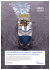More information

### New... - Cranel Imaging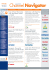More information

### Emeryville Advanced Imaging Referral Form Back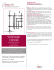More information

### RIMI Capabilities Patient Brochure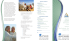More information

### Imaging Unlimited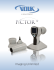More information

### Light Sheet Fluorescence Microscopy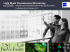More information

### Archiving 2010 Call for Papers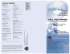More information

### Light-sheet microscopy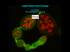More information

### Mindray DC-7 Technology Story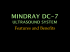More information

### M-OSRP 2015 Annual Meeting Agenda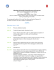More information